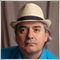# iMAOnArray45

Hi everybody!

What does mean items, Total, and elements in  iMAOnArray?

```double  iMAOnArray(

double       array[],          // array with data
int          total,            // number of elements
int          ma_period,        // MA averaging period
int          ma_shift,         // MA shift
int          ma_method,        // MA averaging method
int          shift             // shift
);```

total

[in]  The number of items to be counted. 0 means the whole array.

Why should I use another value than 0 ?

And why when I use another value than zero, it turnes different values in different terminal (with Same data)?11039

Stable_Profit #Any suggestion?

It is a question of understanding English.

An array is a collection of elements. If an array is of size 100, then there are 100 elements in the array.

If you only want to calculate the moving average for only 50 of those 100 elements, then the total number of elements to be processed is 50.45

Fernando Carreiro #:

It is a question of understanding English.

An array is a collection of elements. If an array is of size 100, then there are 100 elements in the array.

If you only want to calculate the moving average for only 50 of those 100 elements, then the total number of elements to be processed is 50.

Thanks

But If I want to calculate MA for 50 elements, I set ma_period on 50.

what is the differences between ma_period and total?

If total is a way to reduce calculation for unneeded parts of an array, why when I use another value than zero, it turns different values in different terminals (with the same data)?11039

Stable_Profit #: But If I want to calculate MA for 50 elements, I set ma_period on 50. what is the differences between ma_period and total?

If total is a way to reduce calculation for unneeded parts of an array, why when I use another value than zero, it turns different values in different terminals (with the same data)?

No, " ma_period " is the period of the moving average, not the number of elements you want to process.

For example, an EMA(5) over 50 elements, would be "ma_period = 5, total = 50".

Please do some research into how moving averages work, especially on EMA which require much more data than the period.27845

Fernando Carreiro #: specially on EMA which require much more data than the period.

Exponential requires 3.45×(Length+1) bars to converge to 1/10 percent. (EMA Period=5 requires 21 bars.)
Moving average - Wikipedia